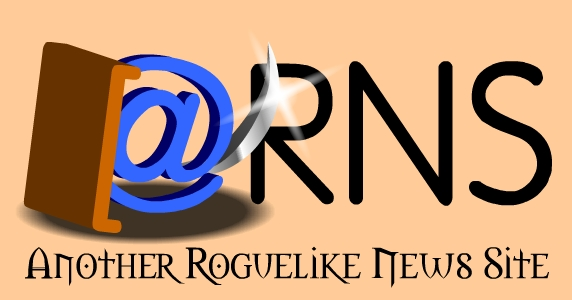| MAIN | NEWS | FAQS | LINKS | ARTICLES | WORKSHOP | Send Mail To:Steve Register

 Field Of Vision - Régis Dubois.
 This article was originally written for Darren Hebden's RLNews ```Written by Régis Dubois the 19/07/99. email: heroicjourneys@altern.org homepage: www.altern.org/heroicjourneys All sources in C. Use them at will! Ok here is another article relating about the famous "line of sight" problem. Why such an article? Because even with all the help provided through the internet I wasn't able to find an easy and efficient solution to solve the problem. So here I'll expose my method. This is a VERY simple method, ok? It means that it is probably slow, that the result isn't very accurate but it is easy to implement and understand. So stop talking and let's start over! << Euh... yes a last thing. Forgive my poor english but I'm french and like lot of you guys know about it, French people aren't famous for their performance in english :) >> Remark: we will use the following structures all through the examples: struct coord { int x,y; }; struct map { int data[line][col]; short visible[line][col]; 0 if invisible, 1 otherwise }; Ok, what we want is to highlight the area around the character, representing what he can see. It means that our character can see every empty spaces or monsters in his line of sight but can not see what is behind walls or closed doors. How to do this? Well we will just throw some rays from the character, in circle motion, so that it covers all what the player can see. Hey! I can hear you scream from here! You think "A circle? So we gonna need to use cos and sin huh?". Well you are right, we need them but we won't let the CPU calculate them at run-time, we will use pre-generated arrays, what we lose in memory, we gain it in speed: const double Pi = 3.1415926535; //need explanation for that? double Cosinus;// array implemented by 5 degrees. 5*72=360 double Sinus;// Why an implementation of 5 degrees? Well, huh, why not? I found it efficient like this but you may arrange it so that it fits your sight range. Let's generate the arrays now: void GenerateTables() { int i; for (i=0;i<72;i++) { Cosinus[i]=cos(i*5*Pi/180); } for (i=0;i<72;i++) { Sinus[i]=sin(i*5*Pi/180); } } I think I don't need to explain these function. Ok what we need now is a function to round a double properly. For example if we have 2,3 we want the function to return us 2 and if we have 2,6 we want the function to return us 3. I checked all the standard libraries in C but couldn't find one. If you know one, please email me! //Round a number to the closest integer int round(double x) { double b; if (modf(x,&b)>0.5) return((int)b+1); else return((int)b); } The next step is to get the co-ordinates of the ray we gonna cast. We will cast it from the player, according to a certain angle. So we have the origin of the line we cast (the player x,y) but we want the co-ordinates of the end of the line. Here is the function that will give them to us: struct coord CastRay(int x,int y,int r, int angle) { struct coord c; c.x=round(x+r*Cosinus[(int)angle/5]); c.y=round(y-r*Sinus[(int)angle/5]); return (c); } Hey guys don't you understand this? This is simple geometry! A small projection. Please note that here r is the radian or length of the ray we cast. It means the sight range. Good we have the co-ordinate of the ray we want to cast, so we have to cast it. We will use the well known Bresenham's line algorithm for this. Here is the line of sight routine, I'll explain it later: int Sgn(int x) { if (x==0) return(0); if (x>0) return(1); if (x<0) return(-1); } // The line of Sight routine. struct Map Los(struct Map m,int x1,int y1,int x2,int y2) { int i,j,dx,dy,sdx,sdy,dxabs,dyabs,x,y,px,py; dx=x2-x1; dy=y2-y1; dxabs=abs(dx); dyabs=abs(dy); sdx=Sgn(dx); sdy=Sgn(dy); x=dyabs/2; y=dxabs/2; px=x1; py=y1; if (dxabs>=dyabs) { for (i=0;i=dxabs) { y-=dxabs; py+=sdy; } px+=sdx; if (m.visible[py][px]==0) //is the square visible? { //no? m.visible[py][px]=1; //no it is PutTile(px,py,m.data[py][px]); //we draw the tile on screen } if (m.data[py][px]!=Empty && m.data[py][px]!=OpenDoor) //if it is neither empty or an opened door, we stop... return(m); } } else { for (i=0;idyabs) { x-=dyabs; px+=sdx; } py+=sdy; if (m.visible[py][px]==0) { m.visible[py][px]=1; PutTile(px,py,m.data[py][px]); } if (m.data[py][px]!=Empty && m.data[py][px]!=OpenDoor) return(m); } } return(m); } The only thing we do here is to cast the ray and checked every square it crosses, if it is empty we light it and carry on further, but if it is a wall we stop. Easy no? Ok it was the tough part, now let's finish by writing the LookAround() function which will cast the 72 rays from the player so that it makes a circle and hence, a field of vision: void LookAround() { struct coord c; int i; for (i=0;i<72;i++) { c=CastRay(p.px,p.py,p.sight,i*5); //we get the coordonates of the line of sight map=Los(map,player.px,player.py,c.x,c.y); }; } And it's done! I know this method isn't the fastest you can find, but it works for sure because I use it in my own Rogue-like project. I hope the explanations were not too dark... If so, feel free to e-mail me: heroicjourneys@altern.org www.altern.org/heroicjourneys Good Luck! Regis. ```